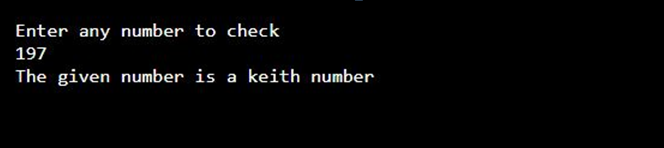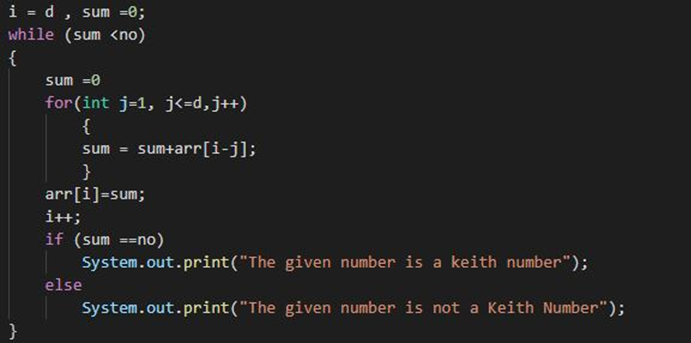# Program to check Keith Number in Java

In this tutorial you will learn about the Program to check Keith Number in Java and its application with practical example.

In this tutorial, we will learn to create a Java Program to check Keith Numbers in Java using Java programming.

## Prerequisites

Before starting with this tutorial we assume that you are best aware of the following Java programming topics:

• Java Operators.
• Basic Input and Output function in Java.
• Class and Object in Java.
• Basic Java programming.
• If-else statements in Java.
• For loop in Java.

## What is Keith number in mathematics?

In mathematics a Keith numberKeith numbers are also called repfigit and it is a positive N-digit integer value  where  Number is greater than 9 such that if we create a series like – Fibonacci( Where each numbers/term in the following series is the sum of the n previous numbers/terms) and this series is formed by the sum of first n terms taken as the integervalue of the number N, then N itself occurs as a term in the series. i.e. 197 is a Keith number as it makes the series asfollows:

1, 9, 7,

1+9+7=17,

9+7+17=33,

7+17+33=57,

17+33+57=107,

33+57+107=197 ->(Keith).

Keith numbers are known as repfigit (repetitive fibonacci-like digit) numbers.

Here: –

N = number of digits of the term.

n = number of terms in the series.

## Java Program to Find Keith Number

In this program we will find the number given by the user is a Keith number or not with the help of Java Program. We will first declare and initializethe required variables for the program. Next, we will prompt the user to input the value in integer format. Later we will check the given value is a Keith number or not.

## OutputIn the above program, we have first declared and initialized a set of variables required in the program.

• no = it will hold entered length.
• no1=  it will replicated value of no1 for digit calculation.
• d=  it will hold the number of digits.
• sum = it will hold the summation of no. of term terms.
• arr[]=  is a array for holding all the terms of series.
• i,j = will hold the numerical value to execute the loop.

Keith number is a term/entity which is repeated on a series when  of a number is break down into the respective digits and add to get the desired number again.

After that take valuesin to an array.Calculating the Keith Number from array we made.In this tutorial we have learn about the Program to check Keith Number in Java and its application with practical example. I hope you will like this tutorial.